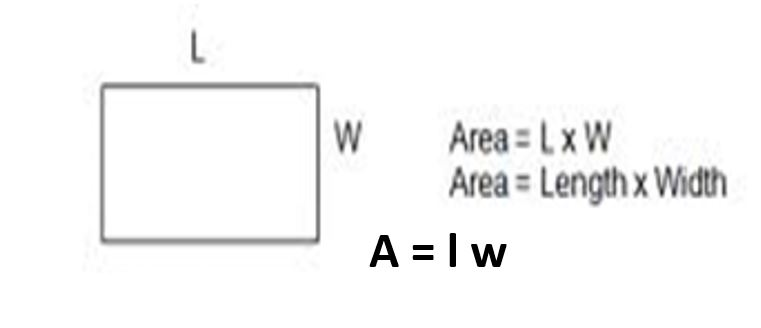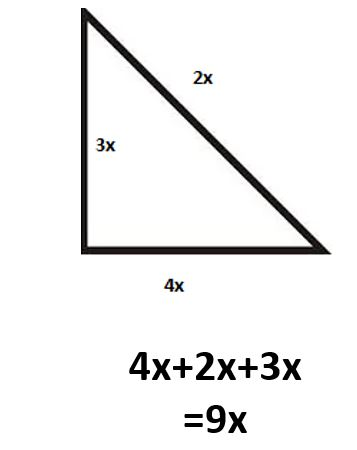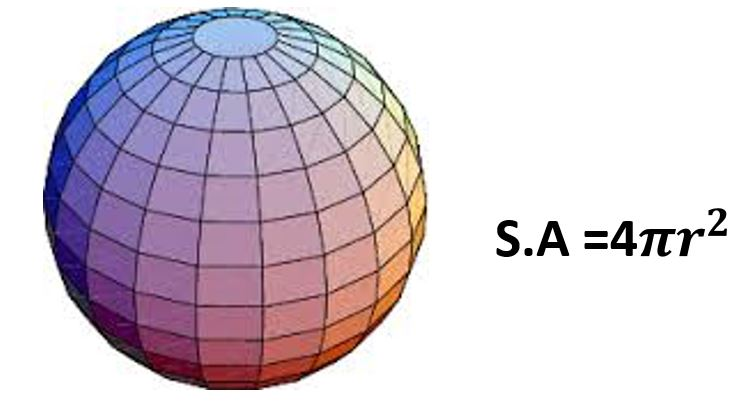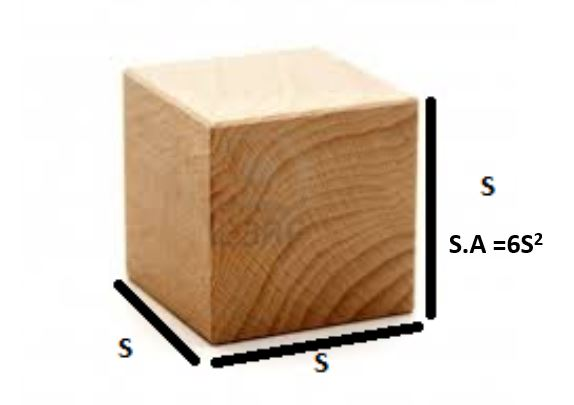Application areas of algebra

Below are some of the areas where algebra has been applied:

#### 1. Formula for calculating area of a Rectangle#### 3. Write an expression for the perimeter of the triangle below#### 4. Formula for calculating  surface area of a sphere#### 5. Formula for calculating  surface area of a Cube